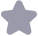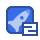### 模拟电子技术基础 第五版 知识点大补贴收藏

2半身体二极管 分失 点按触型 并l圾材料{磕二权管 3按结构}面接阚型 二极 平面型 米3号 正一簧 正 负 米二,伏安特性 乙正向弱性 计 iBr> t UD on 兴注意 )通二营只工作在2区; (1)正向将性 (2)击穷并不意味着报坏 Uon:开启电压 (3)二落正向电流和反向电流 UD:(二极管)马通电压 均不能过大,则会烧管,正 (2)向性 向接法必须串联限流电阻 I5:反向饱和电流I5≈0(4) T 少UD (2)反向击资性 S UBR:反间击穿电压 主要参教 IF UR Ir fM 兴四等效电路 关理想二极管 折 U20 导通LD=0路 儿≤0 截 止工D=0路 L 正员 关2.并关等效电路 L>Uon导通Ub=Uon=i 0.7S 0.2C72 u≤Un截止J=0开路 0 正 负 例.已知U=UD2=07V,=58inWt(),要画波书 十 十 R D 醉:L>+a7VD,导通D2都止0=+0.7V <-0.7V本止D2导通0=-0,7V ≤比≤07VD截止D森止=tL D.7V SV 双向限幅 L/4 D.7 少t D.7V 五狼压二极官 关工作在衣向去穿区 1试安特性 0,为2,工作条件 反向电压UB lgI3≤x3 3.主要参数(课本20-21页) △UL △ 例1已知飞=5U2=10VU1=20VUD=UD2=D7v 中U值 R 十 十 十 十 十 Dg4本30 (a) 7 b) △U六 UoE U b1=5 Uo=U3+032=15V 识也路小,B完全小, 例2已知U1=20V18=1mAPm=200mM R2=50DUk=6V屯RL=100DR2=/k时的山值 十 R D I- mA R-k 1=P8m=3,3mA )2∠=D.A时设截止Ub=2 R+Rz=3.3V∠U 设D些,∴D止Up=Ub=3y 2)R=1kD时设截止U6==13V ,到断电 设D压 ID2=1R-I=业n4k-=22mA L 13<13<13m∴D3德玉U=u=6V 课本52员自训融四 兴13晶体三极管 结构徊炎型 JN]集电区发射区高彬杂 基区基区:薄低集 发射区葆电区:面大,低杂 C NPN PNP 二电流放大作用 放大条件:正偏几反偏 基叔电流集电极电流 出 IE=IB+Ic 入R b le N BB F Rc发角电流 ∨cc 流子运动上圈) 2.电流分配关系 1 B IE=IB+Ic 正PE>7B NPM PNP 3放大福教 8= IB 直流电流放大信教→F≈P沙 △LL △L 以后用B,不再加以区分 流改大信教 例 NPN型两入一出为 .Im N5ImA Ic_5 50 5mA B 共射特曲线 UCE=UBE ⊥B B4 B LB UBE E Rc (2) Rb 1 B=⊥B Vcc IB= IBI 63 ICED 28=0 (1) 输入特性曲线 (截区:e反偏]反偏 =fUB)uE:序 E IcE≈0 关2输特性曲线 NPN:UE≤ Uon UCE>UBE i=f(uaB)l12-数 (2放大区:正偏J反偏 (1)(2) 见方测→ 将点:∫ Ic:BlB (3)饱区:把正偏正偏 UBE=Uen =fa. 条件 SNPN: UBE>Uon UCE <UBE 条件NN: UBE >Uon PNP:UkB>Um比kE>UBE UCE >UBE 特点:①UBE CO7V Si Io.>v Gre PNP: UEB >Uon UcELUBE I《PB UeE=UB-UE UCE=UC-UE ②UE=UcEs<Uon NPN: UC7UB7UE 砝管深饱孙 你加管压降 PNP: UE7UB7Uc UCES=D2-0.3V b间电压差大过Uon CE=UBE界饱加 例.判断状态 12V 。+bV D.7V 十V +53V 0 +53V ()放大状态(b)坏(C)临界饱加 了>0.7 课本54页题1.9 2 (与的差D7V或 b(中间电压) s(装 Ue zUc PNP需头指内 Uc>Ue NPN) 五温度影响 T UBE√ c 第二章基本放大电路 2放大的掘念及放大电路性能挡标 念 1的交流量路大的交说量 直流能量 器 能量转化 交流能量 直流电对室流放大电路至关重要 二性能指标图课本5图21>都是交 1放大倍数A 米Au=A丝=电压放大培数Aa=￥转阻 (电阻放大倍数 Aii= Ai 电流放大信数 A 比-D 转移电号 关掄入电阻R (电马放大倍数) L Ri→I→对信号源系取电流越 米3输出电阻Ro )加压玄载或流法 R RL Uc或短路 2)实验测试 逊R开路时的U,接入RU R。=(-1)R∠ R越、电路蒂负裁能力越强,U越稳定 4通频带fAo lAL At OTo/Awni 5最大不真输出电压,最大输出功率与效 22基本共射放大电路工作原理 电路组成1 IBa IRc 丁:核 R toh A TUcO vc:能量来源 UBE2_ uo CC Rc:电流的变化转化为电压变化 V8.Rb:Je正偏 Vc.Rc.VB.VRb:反偏 并二态平作点Q U=0时T的四个量 UBEQ I662 Ica UcE为Q点 UBEQ SO.7V S Ica=blB D2v Ge I B2= VBB-UBER UCEQ=Vcc -IcaC Rb 三,形分斩 x=70VB→→1 BaxpsIce→>U(ca V8+L→>B+>1ca+i→>U+u LB Lo写0 →>t :纯交流 B 4实直流并存瞬时值 c e C 1B:直流量 Llce /ild o b:女流有效值

...展开详情

抢沙发
一个资源只可评论一次，评论内容不能少于5个字
qq_41745782关注 私信 TA的资源
上传资源赚积分,得勋章
最新推荐模拟电子技术基础 第五版 知识点大补贴 50积分/C币 立即下载
1/53试读已结束，剩余37页未读...

50积分/C币 立即下载 ＞